# Fisseha Berhane, PhD

#### Data Scientist

Picking a good threshold value in binary classification problems is often challenging. The cut-off value we may choose can vary based on the business problem we are solving. If we're more concerned with having a high specificity or low false positive rate, we pick the threshold that maximizes the true positive rate while keeping the false positive rate really low. On the other hand, if we're more concerned with having a high sensitivity or high true positive rate, we pick a threshold that minimizes the false positive rate but has a very high true positive rate.

To evaluate the performance of a model or to compare models, rather than considering metrics such as accuracy, sensitivity, specificity, precision or F-1 score, it is better to use measures that do not depend on a single cut-off value. A Receiver Operator Characteristic curve (ROC curve) and Precision-Recall Curve are what we are going to discuss in this blog post.

An ROC curve is the most commonly used tool for comparing models or to evaluate a model performance. It does not depend on a single cut-off value. To create an ROC curve, the sensitivity, or true positive rate of the model, is shown on the y-axis and the false positive rate, or one minus specificity, is given on the x-axis. The ROC curve always starts at the point (0, 0) and this corresponds to a threshold value of 1. If you have a threshold of 1, you will not catch any positive cases, or have a sensitivity of 0. But you will correctly label all the negative cases, meaning you have a false positive rate of 0. The ROC curve always ends at the point (1,1), which corresponds to a threshold value of 0. If you have a threshold of 0, you'll catch all of the positive cases, or have a sensitivity of 1, but you'll label all of the negative cases as positive cases too, meaning you have a false positive rate of 1. The threshold decreases as you move from (0, 0) to (1, 1). The ROC curve captures all thresholds simultaneously. The higher the threshold, or closer to (0, 0), the higher the specificity and the lower the sensitivity. The lower the threshold, or closer to (1,1), the higher the sensitivity and lower the specificity.

Precision-Recall Curve is another tool that does not depend on a single threshold value. In this case, the precision is shown on the y-axis while the sensitivity, also called recall, is shown on the x-axis. The Precision-Recall starts at (0,1) and as will be shown below when the data is imbalanced using the ROC Curve could be misleading and Precision-Recall curve is more informative.

Let's generate datasets and build lasso logistic regression using grid search with cross-validation for hyper-parameter tuning. First, we will generate balanced data, where the two classes have about equal counts, and plot the ROC and Precision-Recall Curves, and culculate the areas under the curves. Next, we will generate imbalanced data where the labels are 98% from one class. Imbalanced data is very common in classification problems but we usually see ROC curves being used to evaluate such models. However, as you will see below, ROC curves are not good tools for imbalanced data.

In :
import numpy as np
from sklearn.linear_model import LogisticRegression
from sklearn.model_selection import train_test_split
from sklearn.metrics import roc_curve
from sklearn.metrics import precision_recall_curve
from sklearn.metrics import average_precision_score
from sklearn.metrics import roc_auc_score
from sklearn.model_selection import GridSearchCV
from sklearn.pipeline import Pipeline
from sklearn.preprocessing import StandardScaler
from sklearn.datasets import make_classification
import collections
import matplotlib.pyplot as plt
%matplotlib inline


## Balanced Data¶

### Generate binary class dataset and split into train/test sets¶

Let's generate 100000 samples with 30 features.

In :
X, y = make_classification(n_samples = 100000, n_features = 30, n_classes = 2, weights = [0.5,0.5], random_state = 1)
X_train, X_test, y_train, y_test = train_test_split(X, y, test_size = 0.3, random_state = 42)


#### Now, let's see the count of the label values¶

In :
collections.Counter(y)

Out:
Counter({0: 50027, 1: 49973})

So, as shown above the labels are more or less balanced.

In :
steps = [('scaler', StandardScaler()),
('logreg', LogisticRegression(penalty = 'l1', solver = 'saga', tol = 1e-6,
max_iter = int(1e6), warm_start = True, n_jobs = -1))]

pipeline = Pipeline(steps)
param_grid = {'logreg__C': np.arange(0., 1, 0.1)}
logreg_cv = GridSearchCV(pipeline, param_grid, cv = 5,  n_jobs = -1)
logreg_cv.fit(X_train, y_train)

Out:
GridSearchCV(cv=5, error_score='raise-deprecating',
estimator=Pipeline(memory=None,
steps=[('scaler', StandardScaler(copy=True, with_mean=True, with_std=True)), ('logreg', LogisticRegression(C=1.0, class_weight=None, dual=False, fit_intercept=True,
intercept_scaling=1, max_iter=1000000, multi_class='warn',
n_jobs=-1, penalty='l1', random_state=None, solver='saga',
tol=1e-06, verbose=0, warm_start=True))]),
fit_params=None, iid='warn', n_jobs=-1,
param_grid={'logreg__C': array([0. , 0.1, 0.2, 0.3, 0.4, 0.5, 0.6, 0.7, 0.8, 0.9])},
pre_dispatch='2*n_jobs', refit=True, return_train_score='warn',
scoring=None, verbose=0)

### What are the best parameter and best score?¶

In :
print ('best score:', logreg_cv.best_score_)
print ('best parameter:',logreg_cv.best_params_)

best score: 0.9167714285714286
best parameter: {'logreg__C': 0.1}


### Fit lasso logistic regression using the best parameter above¶

In :
scaler = StandardScaler()
scaler.fit(X_train)
X_train_scaled = scaler.transform(X_train)
logreg = LogisticRegression(penalty = 'l1', solver = 'saga', tol = 1e-6,  max_iter = int(1e6),
warm_start = True, C = logreg_cv.best_params_['logreg__C'])
logreg.fit(X_train_scaled, y_train)

Out:
StandardScaler(copy=True, with_mean=True, with_std=True)
Out:
LogisticRegression(C=0.1, class_weight=None, dual=False, fit_intercept=True,
intercept_scaling=1, max_iter=1000000, multi_class='warn',
n_jobs=None, penalty='l1', random_state=None, solver='saga',
tol=1e-06, verbose=0, warm_start=True)

### Lasso can be used for feature selection. Let's plot the coefficients to see which features have been selected¶

In :
lasso_coef = logreg.coef_.reshape(-1,1)
plt.figure(figsize = (20,10))
plt.plot([0,29],[0,0])
_ = plt.plot(range(30), lasso_coef, linestyle='--', marker='o', color='r')
_ = plt.xticks(range(30), range(30), rotation=60)
_ = plt.ylabel('Coefficients')
plt.xlabel('Features', fontsize = 16)
plt.ylabel('Coefficients', fontsize = 16)
plt.xticks(size = 18)
plt.yticks(size = 18)
plt.title('Feature Coefficients from Lasso Logistic Regression', fontsize = 28)
plt.show();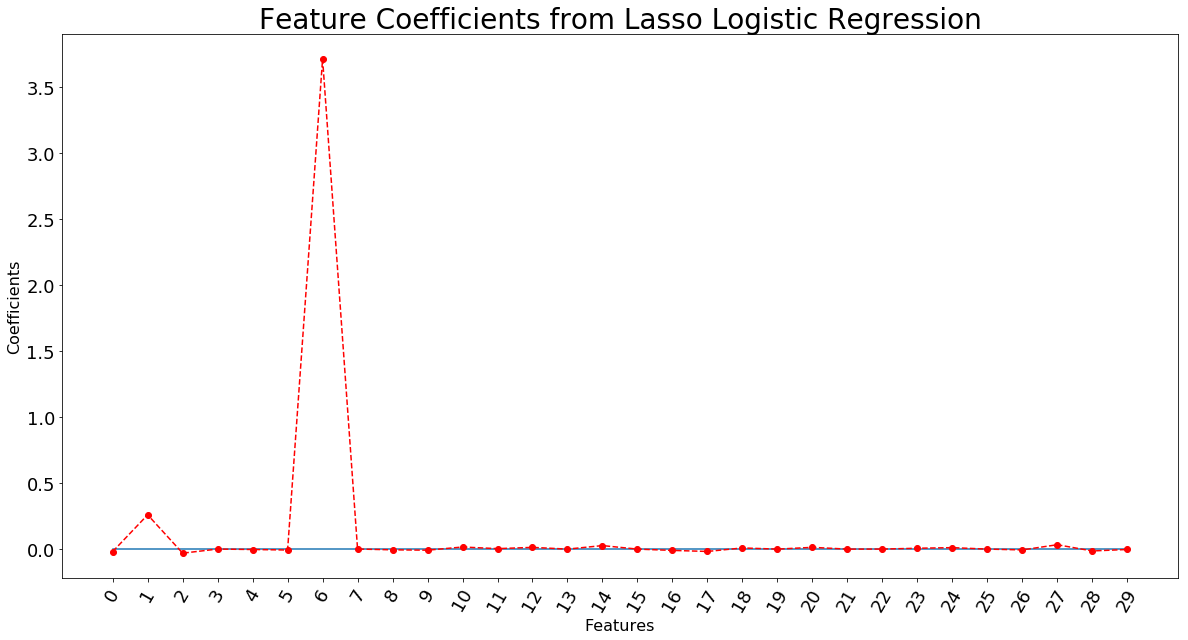### Make predictions using the test dataset¶

In :
X_test_scaled = scaler.transform(X_test)
y_pred_prob = logreg.predict_proba(X_test_scaled)[:,1]  # return probabilities for the positive outcome only


### Plot ROC Curve¶

The dotted blue line is the baseline.

In :
fpr, tpr, thresholds = roc_curve(y_test, y_pred_prob)
plt.figure(figsize = (20,10))
plt.plot([0, 1], [0, 1], linestyle = '--')
plt.plot(fpr, tpr)
plt.xlabel('False Positive Rate', fontsize = 16)
plt.ylabel('True Positive Rate', fontsize = 16)
plt.xticks(size = 18)
plt.yticks(size = 18)
plt.title('Lasso Logistic Regression ROC Curve', fontsize = 28)
plt.show();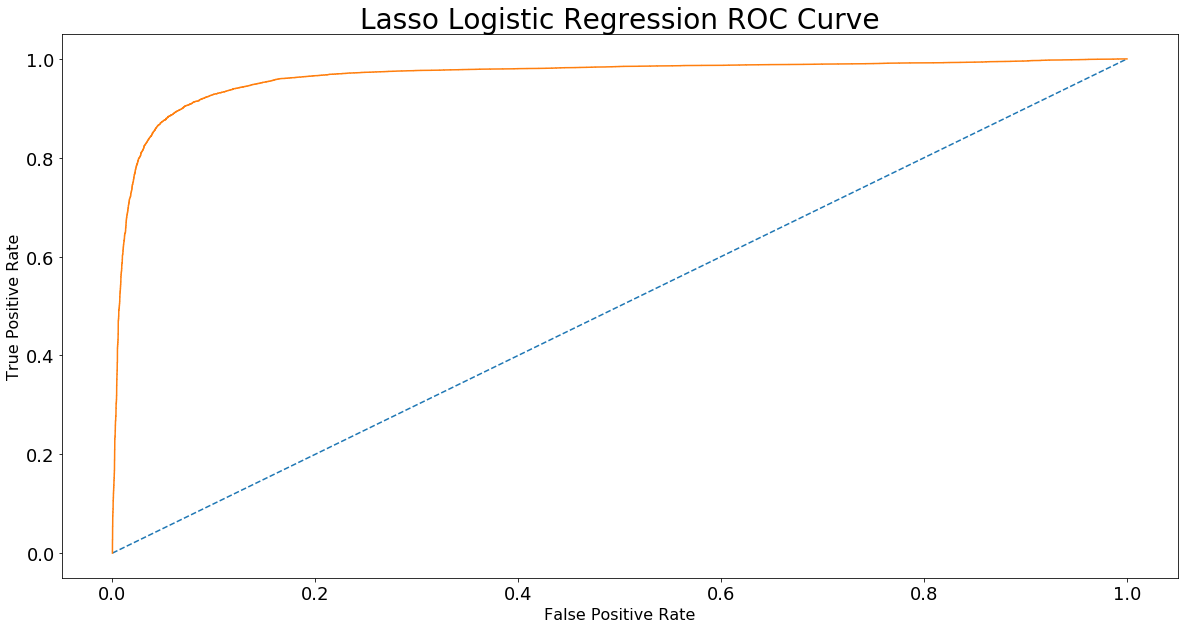### What is the area under the curve?¶

In :
round(roc_auc_score(y_test, y_pred_prob), 2)

Out:
0.96

### Now, plot the Precision-Recall Curve and calculate the area under the curve¶

The dotted orange line is the baseline

In :
precision, recall, thresholds = precision_recall_curve(y_test, y_pred_prob)
plt.figure(figsize = (20,10))
plt.plot(recall, precision)
plt.plot([0, 1], [0.5, 0.5], linestyle = '--')
plt.xlabel('Recall', fontsize = 16)
plt.ylabel('Precision', fontsize = 16)
plt.xticks(size = 18)
plt.yticks(size = 18)
plt.title('Lasso Logistic Regression Precision-Recall Curve', fontsize = 28)
plt.show();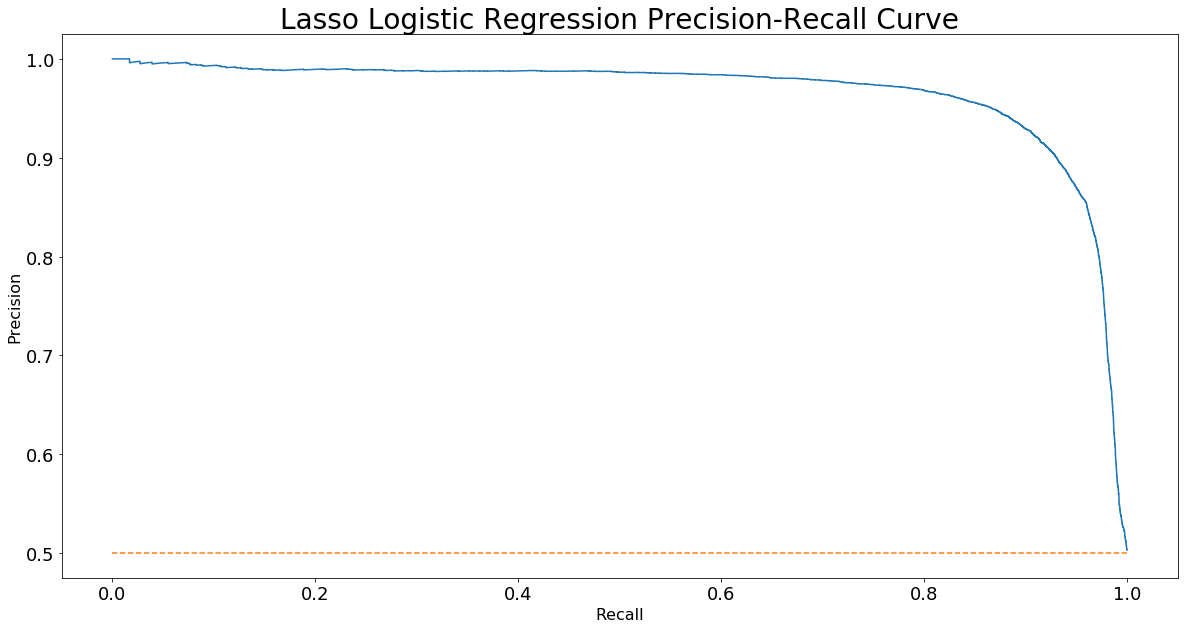### What is the area under the Precision-Recall curve?¶

In :
round(average_precision_score(y_test, y_pred_prob), 2)

Out:
0.97

## Imbalanced Data¶

### Generate binary class dataset and split into train/test sets¶

Let's generate 100000 samples with 30 features.

In :
X, y = make_classification(n_samples = 100000, n_features = 30, n_classes = 2, weights = [0.99,0.01], random_state = 1)
X_train, X_test, y_train, y_test = train_test_split(X, y, test_size = 0.3, random_state = 42)


### Now, let's see the count of the label values¶

In :
collections.Counter(y)

Out:
Counter({0: 98499, 1: 1501})

So, as shown above the labels are imbalanced (about 98% of them are zeros)

In :
steps = [('scaler', StandardScaler()),
('logreg', LogisticRegression(penalty = 'l1', solver = 'saga', tol = 1e-6,
max_iter = int(1e6), warm_start = True, n_jobs = -1))]

pipeline = Pipeline(steps)
param_grid = {'logreg__C': np.arange(0., 1, 0.1)}
logreg_cv = GridSearchCV(pipeline, param_grid, cv = 5,  n_jobs = -1)
logreg_cv.fit(X_train, y_train)

Out:
GridSearchCV(cv=5, error_score='raise-deprecating',
estimator=Pipeline(memory=None,
steps=[('scaler', StandardScaler(copy=True, with_mean=True, with_std=True)), ('logreg', LogisticRegression(C=1.0, class_weight=None, dual=False, fit_intercept=True,
intercept_scaling=1, max_iter=1000000, multi_class='warn',
n_jobs=-1, penalty='l1', random_state=None, solver='saga',
tol=1e-06, verbose=0, warm_start=True))]),
fit_params=None, iid='warn', n_jobs=-1,
param_grid={'logreg__C': array([0. , 0.1, 0.2, 0.3, 0.4, 0.5, 0.6, 0.7, 0.8, 0.9])},
pre_dispatch='2*n_jobs', refit=True, return_train_score='warn',
scoring=None, verbose=0)

### What are the best parameter and best score?¶

In :
print ('best score:', logreg_cv.best_score_)
print ('best parameter:',logreg_cv.best_params_)

best score: 0.9859285714285714
best parameter: {'logreg__C': 0.30000000000000004}


### Fit lasso logistic regression using the best parameter above¶

In :
scaler = StandardScaler()
scaler.fit(X_train)
X_train_scaled = scaler.transform(X_train)
logreg = LogisticRegression(penalty = 'l1', solver = 'saga', tol = 1e-6,  max_iter = int(1e6),
warm_start = True, C = logreg_cv.best_params_['logreg__C'])
logreg.fit(X_train_scaled, y_train)

Out:
StandardScaler(copy=True, with_mean=True, with_std=True)
Out:
LogisticRegression(C=0.30000000000000004, class_weight=None, dual=False,
fit_intercept=True, intercept_scaling=1, max_iter=1000000,
multi_class='warn', n_jobs=None, penalty='l1', random_state=None,
solver='saga', tol=1e-06, verbose=0, warm_start=True)

### Let's plot the coefficients to see which features have been selected¶

In :
lasso_coef = logreg.coef_.reshape(-1,1)
plt.figure(figsize = (20,10))
plt.plot([0,29],[0,0])
_ = plt.plot(range(30), lasso_coef, linestyle='--', marker='o', color='r')
_ = plt.xticks(range(30), range(30), rotation=60)
_ = plt.ylabel('Coefficients')
plt.xlabel('Features', fontsize = 16)
plt.ylabel('Coefficients', fontsize = 16)
plt.xticks(size = 18)
plt.yticks(size = 18)
plt.title('Feature Coefficients from Lasso Logistic Regression', fontsize = 28)
plt.show();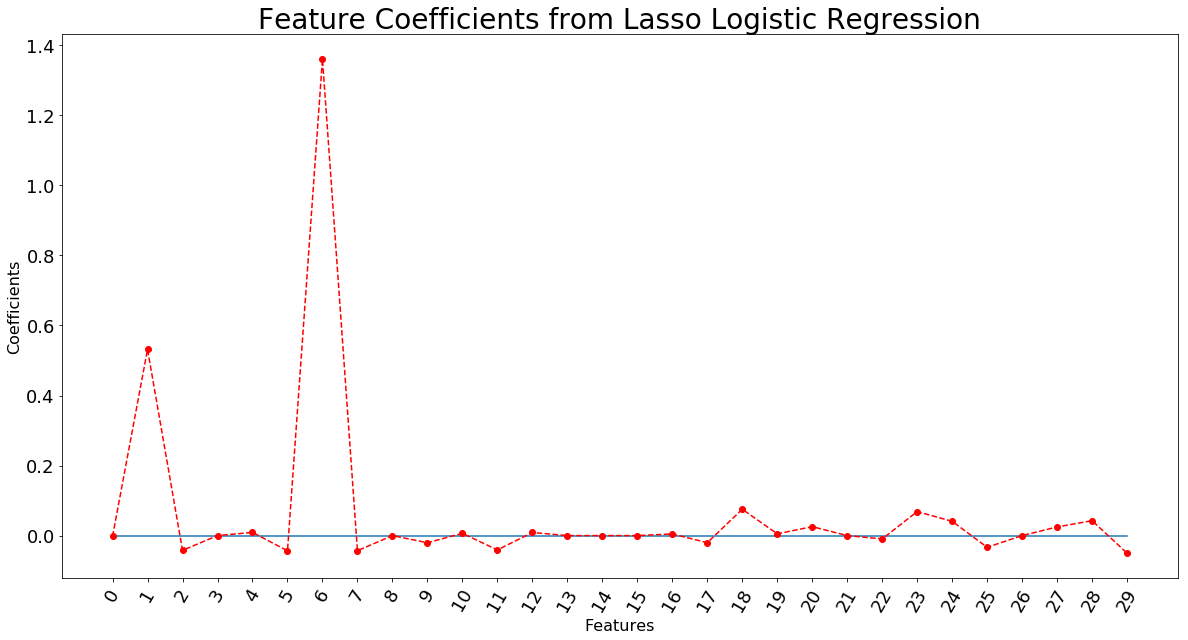### Make predictions using the test dataset¶

In :
X_test_scaled = scaler.transform(X_test)
y_pred_prob = logreg.predict_proba(X_test_scaled)[:,1]  # return probabilities for the positive outcome only


### Plot ROC Curve¶

The dotted blue line is the baseline

In :
fpr, tpr, thresholds = roc_curve(y_test, y_pred_prob)
plt.figure(figsize = (20,10))
plt.plot([0, 1], [0, 1], linestyle = '--')
plt.plot(fpr, tpr)
plt.xlabel('False Positive Rate', fontsize = 16)
plt.ylabel('True Positive Rate', fontsize = 16)
plt.xticks(size = 18)
plt.yticks(size = 18)
plt.title('Lasso Logistic Regression ROC Curve', fontsize = 28)
plt.show();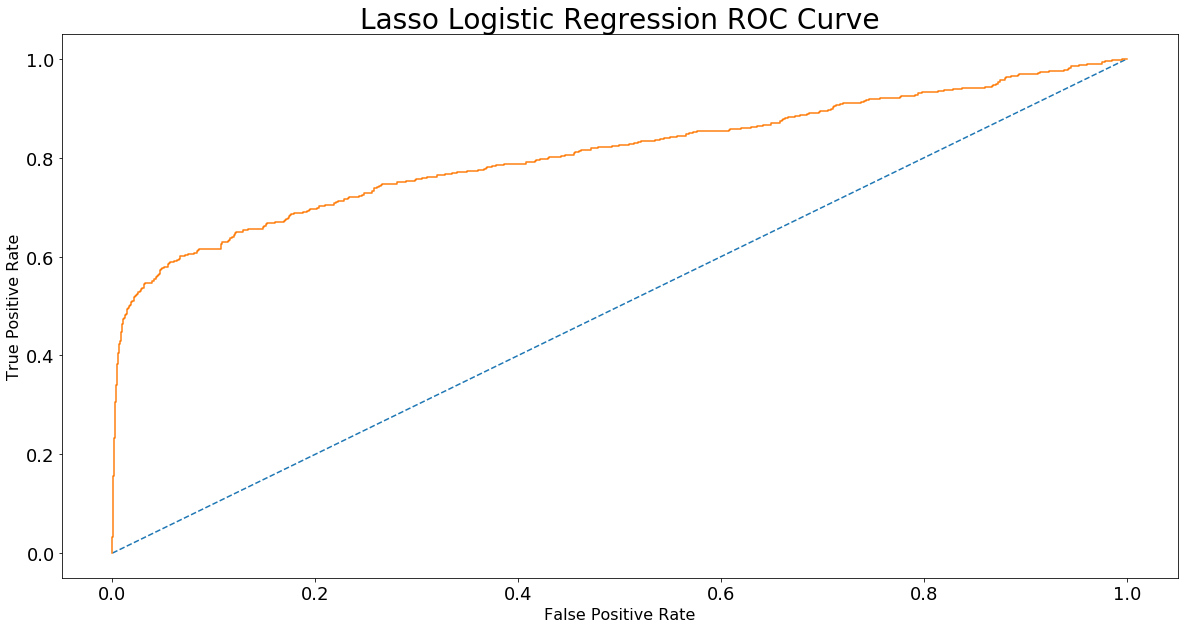In :
round(roc_auc_score(y_test, y_pred_prob), 2)

Out:
0.81

### Now, plot the Precision-Recall Curve and calculate the area under the curve¶

The dotted blue line is the baseline

In :
precision, recall, thresholds = precision_recall_curve(y_test, y_pred_prob)
plt.figure(figsize = (20,10))
plt.plot([0, 1], [0.01/0.98, 0.01/0.98], linestyle = '--')
plt.plot(recall, precision)
plt.xlabel('Recall', fontsize = 16)
plt.ylabel('Precision', fontsize = 16)
plt.xticks(size = 18)
plt.yticks(size = 18)
plt.title('Lasso Logistic Regression Precision-Recall Curve', fontsize = 28)
plt.show();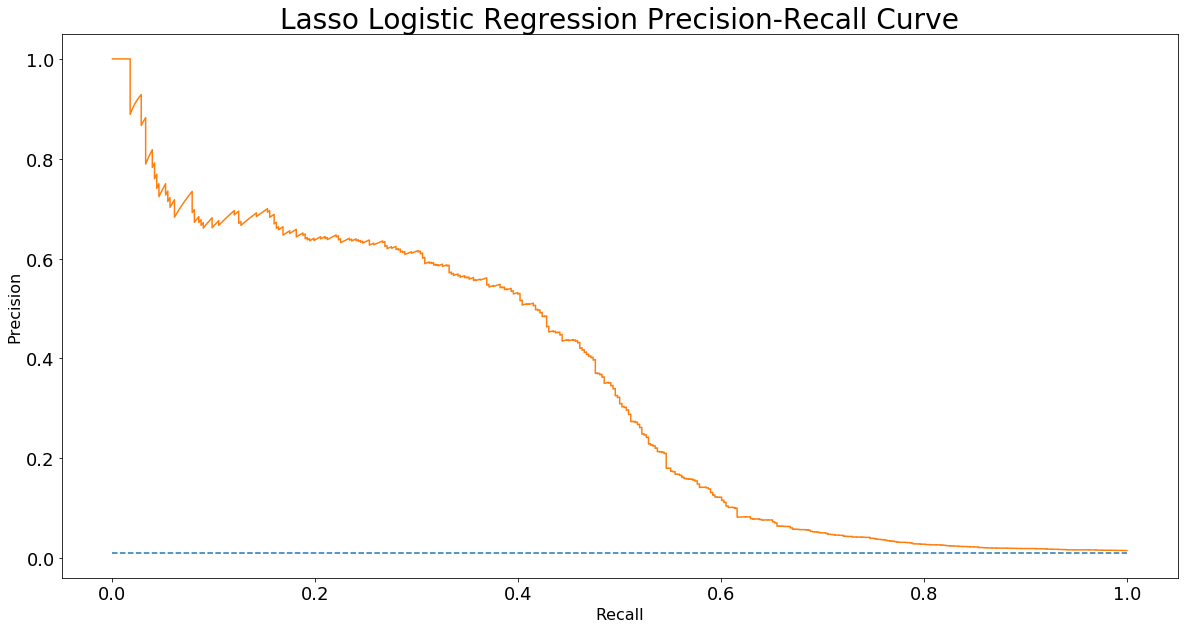In :
round(average_precision_score(y_test, y_pred_prob), 2)

Out:
0.35

From the results above, when the data is imbalanced, the area under the ROC curve and the area under the Precision-Recall curve are very different and the Precision-Recall curve is more informative than the ROC curve.

## Summary¶

Even if ROC curve and area under the ROC curve are commonly used to evaluate model performance with balanced and imbalanced datasets, as shown in this blog post, if your data is imbalanced, Precision-Recall curve and the area under that curve are more informative than the ROC curve and area under the ROC curve. Actually, ROC curve could be misleading for binary classification problems with imbalanced data.